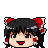# POJ 2823. Sliding Window

### Brief description:

。。維護一個寬度為 k 的滑動窗口。。分別統計所有位置的最大值和最小值。。

# SRM 491

500 :

1：數字範圍在[1，N]之間且兩兩不相同。
2：對面數字和相等且 >= k。
3：經過旋轉相同的篩子被看作是等價的。

（room 里有人用DP?.. 是怎麼DP的呢？）

1000 :

#include
using namespace std;

int Cn3(int n){
if (n < 3) return 0;
return n * (n-1) * (n-2) / 6;
}

class FoxMakingDiceEasy{
public:
int theCount(int n, int k){
int res = 0, MAX = n * 2 - 5;
// i: 為了計算，枚舉正反兩面的數字和 - 1 的值。
for (int i=k-1;i
#include
#include
using namespace std ;

class BottlesOnShelf
{
public :
long long  gcd( long long a , long long b ) { if ( b == 0 ) return a ; return gcd(b , a%b) ; }
long long  lcm( long long a , long long b ) { long long c = gcd(a , b) ; return a * b / c ; }

int getNumBroken(int N, vector  L, vector  R, vector  D)
{
int M = L.size() , p , ll , rr , ans ;
long long w , c ;

ans = 0 ;
for ( int i = 1 ; i < (1 << M) ; i ++ )
{
p = 0 ;
for ( int j = 0 ; j < M ; j ++ ) if ( ( i >> j ) & 1 ) p ++ ;

ll = 1 ; rr = N ; w = -1 ;
for ( int j = 0 ; j < M ; j ++ ) if ( ( i >> j ) & 1 )
{
if ( L[j] > ll ) ll = L[j] ;
if ( R[j] < rr ) rr = R[j] ;
if ( w == -1 ) w = D[j] ; else w = lcm(w , (long long)D[j]) ;
}

c = (long long)(rr / w) - (long long)((ll-1) / w) ;
if ( p % 2 == 1 ) ans += c ; else ans -= c ;
}
return ans ;
}
} ;



# Nim Game

### 序

Nim Game問題是博弈問題中的重頭戲，也是現在信息學競賽中考察最多的。Nim Game往往與xor運算相聯繫，同時又引出了很多的分支，最經典的是Nim-k、anti-Nim、Staircase Nim、take&break四個拓展問題。此外還有Misère Nim，概率問題。ゆっくり読んでください …

# 《毛澤東的讀書生涯》書摘 …

（ … 有所思，摘之，讀之亦覺雋永，假時，竟摘錄全章… 粘至此處願與諸君共饗 …）
–GMT +8 2010 Dec 6th 17:00 於一校區圖書館 。。。

### 毛澤東和他的老師ゆっくり読んでください …